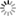##### Personal tools
You are here: Home Mathematics Tools Software

# Mathematics Tools Software

### - The Python Math Library

The Python math library provides us with access to some common math functions and constants in Python that we can use throughout our code to perform more complex math calculations. The library is a built-in Python module, so you don't need any installation to use it. Here are some examples.

• import math
• math.pi
• math.exp(x)

### - SymPy

SymPy (https://www.sympy.org/en/index.html) is a Python library for symbolic mathematics. It aims to become a full-featured computer algebra system (CAS) while keeping the code as simple as possible in order to be comprehensible and easily extensible. SymPy is written entirely in Python.

SymPy is…

• Free: Licensed under BSD, SymPy is free both as in speech and as in beer.
• Python-based: SymPy is written entirely in Python and uses Python for its language.
• Lightweight: SymPy only depends on mpmath, a pure Python library for arbitrary floating point arithmetic, making it easy to use.
• A library: Beyond use as an interactive tool, SymPy can be embedded in other applications and extended with custom functions.

[More to come ...]

##### Document Actions